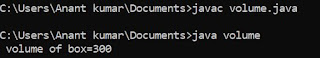## Tuesday, 10 April 2018

### Program to check input number is negative or positive in c

Here we are going to write a program to check a input number is negative or positive .Here first of all we have to declare a variable and then input the values of variable from the user and then we have to check the condition for the negative or positive. These types of program totally depends upon the condition of the program. Here condition may be used as per the mathematical property of variables.Now we going to write the program.

/*number is negative or positive*/
# include <stdio.h>
void main ()
int a;
clrscr();
printf ("\n Enter a number:");
scanf ("%d",& a);
if (a>0)
printf (" a is positive ");
else
printf (" a is negative ");
getch();
}

Here as we written the program we seen that we use a condition for check the positive or negative of a number which is a>0 .It means that when you are writing any program we have use condition for check the value .As example if write program for finding even or odd of a number then we have to firstly know how to check a even or odd number which we are going to discuss in the next blog.

1.2.